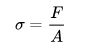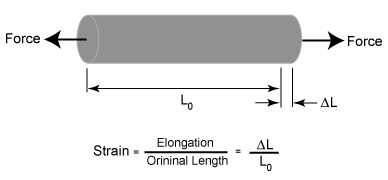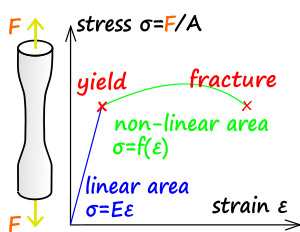### Some Important Concepts in Mechanics of Material

Mechanics of material, also known as strength of material, is a subject which deals with the behavior of solid objects subject to stresses and strains. The complete theory began with the consideration of the behavior of one and two-dimensional members of structures, whose states of stress can be approximated as two dimensional, and was then generalized to three dimensions to develop a more complete theory of the elastic and plastic behavior of materials. An important founding pioneer in mechanics of materials was Stephen Timoshenko.
The study of mechanics of material often refers to various methods of calculating the stresses and strains in structural members, such as columns, beams, and shafts. The methods employed to predict the response of a structure under loading and its susceptibility to various failure modes takes into account the properties of the materials such as its yield strength, ultimate strength, Young's modulus, and Poisson's ratio; in addition, the mechanical element's macroscopic properties (geometric properties), such as its length, width, thickness, boundary constraints and abrupt changes in geometry such as holes are considered.Transverse loading — Forces applied perpendicular to the longitudinal axis of a member. Transverse loading causes the member to bend and deflect from its original position, with internal tensile and compressive strains accompanying the change in curvature of the member. Transverse loading also induces shear forces that cause shear deformation of the material and increase the transverse deflection of the member.

Axial loading — The applied forces are collinear with the longitudinal axis of the member. The forces cause the member to either stretch or shorten.

Torsional loading — Twisting action caused by a pair of externally applied equal and oppositely directed force couples acting on parallel planes or by a single external couple applied to a member that has one end fixed against rotation.

Stress terms

Uniaxial stress is expressed bywhere F is the force [N] acting on an area A [m2]. The area can be the undeformed area or the deformed area, depending on whether engineering stress or true stress is of interest.

Compressive stress (or compression) is the stress state caused by an applied load that acts to reduce the length of the material (compression member) along the axis of the applied load, it is, in other words, a stress state that causes a squeezing of the material. A simple case of compression is the uniaxial compression induced by the action of the opposite, pushing forces. Compressive strength for materials is generally higher than their tensile strength. However, structures loaded in compression are subject to additional failure modes, such as buckling, that are dependent on the member's geometry.

/ is the stress state caused by an applied load that tends to elongate the material along the axis of the applied load, in other words, the stress caused by pulling the material. The strength of structures of equal cross-sectional area loaded in tension is independent of the shape of the cross-section. Materials loaded in tension are susceptible to stress concentrations such as material defects or abrupt changes in geometry. However, materials exhibiting ductile behavior (most metals for example) can tolerate some defects while brittle materials (such as ceramics) can fail well below their ultimate material strength.

Shear stress is the stress state caused by the combined energy of a pair of opposing forces acting along parallel lines of action through the material, in other words, the stress caused by faces of the material sliding relative to one another. An example is cutting paper with scissors or stresses due to torsional loading.

Strength terms

Mechanical properties of materials include yield strength, tensile strength, fatigue strength, crack resistance, and other characteristics.

Yield strength is the lowest stress that produces a permanent deformation in a material. In some materials, like aluminum alloys, the point of yielding is difficult to identify, thus it is usually defined as the stress required to cause a 0.2% plastic strain. This is called a 0.2% proof stress.

Compressive strength is a limit state of compressive stress that leads to failure in a material in the manner of ductile failure (infinite theoretical yield) or brittle failure (rupture as the result of crack propagation, or sliding along a weak plane.

Tensile strength or ultimate tensile strength is a limit state of tensile stress that leads to tensile failure in the manner of ductile failure (yield as the first stage of that failure, some hardening in the second stage and breakage after a possible "neck" formation) or brittle failure (sudden breaking in two or more pieces at a low-stress state). The tensile strength can be quoted as either true stress or engineering stress, but engineering stress is the most commonly used.

Fatigue strength is a measure of the strength of a material or a component under cyclic loading and is usually more difficult to assess than the static strength measures. Fatigue strength is quoted as stress amplitude or stress range (), usually at zero mean stress, along with the number of cycles to failure under that condition of stress.

Impact strength is the capability of the material to withstand a suddenly applied load and is expressed in terms of energy. Often measured with the Izod impact strength test or the Charpy impact test, both of which measure the impact energy required to fracture a sample. Volume, modulus of elasticity, distribution of forces, and yield strength affect the impact strength of a material. In order for a material or object to have a high impact strength, the stresses must be distributed evenly throughout the object. It also must have a large volume with a low modulus of elasticity and a high material yield strength.

Strain terms:The deformation of the material is the change in geometry created when stress is applied ( as a result of applied forces, gravitational fields, accelerations, thermal expansion, etc.). Deformation is expressed by the displacement field of the material.

Strain or reduced deformation is a mathematical term that expresses the trend of the deformation change among the material field. Strain is the deformation per unit length. In the case of uniaxial loading, the displacements of a specimen (for example a bar element) lead to a calculation of strain expressed as the quotient of the displacement and the original length of the specimen. For 3D displacement fields, it is expressed as derivatives of displacement functions in terms of a second-order tensor (with 6 independent elements).

Deflection is a term to describe the magnitude to which a structural element is displaced when subject to an applied load.

Stress-strain relationsElasticity is the ability of a material to return to its previous shape after stress is released. In many materials, the relation between applied stress is directly proportional to the resulting strain (up to a certain limit), and a graph representing those two quantities is a straight line.

The slope of this line is known as Young's modulus or the "modulus of elasticity." The modulus of elasticity can be used to determine the stress-strain relationship in the linear-elastic portion of the stress-strain curve. The linear-elastic region is either below the yield point, or if a yield point is not easily identified on the stress-strain plot it is defined to be between 0 and 0.2% strain and is defined as the region of strain in which no yielding (permanent deformation) occurs.

Plasticity or plastic deformation is the opposite of elastic deformation and is defined as unrecoverable strain. Plastic deformation is retained after the release of the applied stress. Most materials in the linear-elastic category are usually capable of plastic deformation. Brittle materials, like ceramics, do not experience any plastic deformation and will fracture under relatively low strain, while ductile materials such as metallics, lead, or polymers will plasticly deform much more before a fracture initiation.